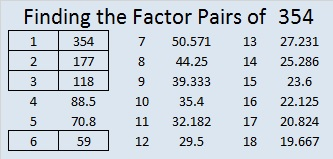# 354 and Level 4

354 is even and thus divisible by 2, so 354 is a composite number. It is also made from three consecutive numbers so it is divisible by 3. Scroll down past the puzzle to see its factors.Print the puzzles or type the factors on this excel file: 12 Factors 2015-01-12

• 354 is a composite number.
• Prime factorization: 354 = 2 x 3 x 59
• The exponents in the prime factorization are 1, 1, and 1. Adding one to each and multiplying we get (1 + 1)(1 + 1)(1 + 1) = 2 x 2 x 2 = 8. Therefore 354 has exactly 8 factors.
• Factors of 354: 1, 2, 3, 6, 59, 118, 177, 354
• Factor pairs: 354 = 1 x 354, 2 x 177, 3 x 118, or 6 x 59
• 354 has no square factors that allow its square root to be simplified. √354 ≈ 18.815